# Creating Formulas to Calculate Values

Once you ve added your data to a worksheet and defined ranges to simplify data references, you can create a formula , or an expression that performs calculations on your data. For example, you can calculate the total cost of a customer s order, figure the average sales for all Wednesdays in the month of January, or find the highest and lowest daily sales for a week, month, or year.

To write an Excel formula, you begin the cell s contents with an equal sign ”when Excel sees it, it knows that the expression following it should be interpreted as a calculation and not text. After the equal sign, you type the formula. For instance, you can find the sum of the numbers in cells C2 and C3 using the formula =C2+C3 . After you have entered a formula into a cell, you can revise it by clicking the cell and then editing the formula in the formula bar. For example, you can change the preceding formula to =C3-C2 , which calculates the difference between the contents of cells C2 and C3.

 Troubleshooting If Excel treats your formula as text, make sure you haven t accidentally put a space before the equal sign. Remember, the equal sign must be the first character!

Typing the cell references for 15 or 20 cells in a calculation would be tedious , but Excel makes it easy to handle complex calculations. To create a new calculation, you click Function on the Insert menu. The Insert Function dialog box appears, with a list of functions , or predefined formulas, from which you can choose.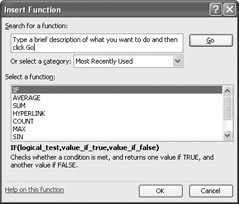The most useful functions in the list are described in the following table:

Item

Description

SUM

Returns the sum of the numbers in the specified cells

AVERAGE

Finds the average of the numbers in the specified cells

COUNT

Finds the number of entries in the specified cells

MAX

Finds the largest value in the specified cells

MIN

Finds the smallest value in the specified cells

Two other functions you might use are the NOW() and PMT() functions. The NOW() function returns the time the workbook was last opened, so the value will change every time the workbook is opened. The proper form for this function is =NOW(); to update the value to the current date and time, just save your work, close the workbook, and then reopen it. The PMT() function is a bit more complex. It calculates payments due on a loan, assuming a constant interest rate and constant payments. To perform its calculations, the PMT() function requires an interest rate, the number of months of payments, and the starting balance. The elements to be entered into the function are called arguments and must be entered in a certain order. That order is written PMT(rate, nper, pv, fv, type ) . The following table summarizes the arguments in the PMT() function:

Argument

Description

rate

The interest rate, to be divided by 12 for a loan with monthly payments

nper

The total number of payments for the loan

pv

The amount loaned (pv is short for present value, or principal)

fv

The amount to be left over at the end of the payment cycle (usually left blank, which indicates 0)

type

0 or 1, indicating whether payments are made at the beginning or at the end of the month (usually left blank, which indicates 0, or the end of the month)

If you wanted to borrow \$20,000 at an 8 percent interest rate and pay the loan back over 24 months, you could use the PMT() function to figure out the monthly payments. In this case, the function would be written =PMT(8%/12, 24, 20000) , which calculates a monthly payment of \$904.55.

You can also add the names of any ranges you ve defined to a formula. For example, if the named range Order1 refers to cells C2 through C6, you can calculate the average of cells C2 through C6 with the formula =AVERAGE(Order1) . If you want to include a series of contiguous cells in a formula but you haven t defined the cells as a named range, you can click the first cell in the range and drag to the last cell. If the cells aren t contiguous, hold down the [CONTROL] key and click the cells to be included. In both cases, when you release the mouse button, the references of the cells you selected appear in the formula.

Another use for formulas is to display messages when certain conditions are met. For instance, Catherine Turner, the owner of The Garden Company, might provide a free copy of a gardening magazine to customers making purchases worth more than \$150. This kind of formula is called a conditional formula, and it uses the IF function. To create a conditional formula, you click the cell to hold the formula and open the Insert Function dialog box. From within the dialog box, you select IF from the list of available functions and then click OK. The Function Arguments dialog box appears.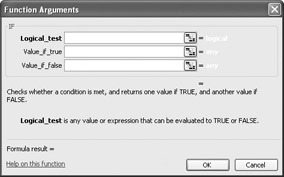When you work with an IF function, the Function Arguments dialog box will have three boxes: Logical_test, Value_if_true, and Value_if_false. The Logical_test box holds the condition you want to check. To check whether the total for an order is greater than \$150, the expression would be SUM(Order1) > 150 .

Now you need to have Excel display messages indicating whether the customer should receive a free magazine. To have Excel print a message from an IF function, you enclose the message in quotes in the Value_if_true or Value_if_false box. In this case, you would type Qualifies for a free magazine! in the Value_if_true box and Thanks for your order! in the Value_if_false box.Once you ve created a formula, you can copy it and paste it into another cell. When you do, Excel will try to change the formula so that it works in the new cells. For instance, in the following graphic, cell D8 contains the formula =SUM(C2:C6) .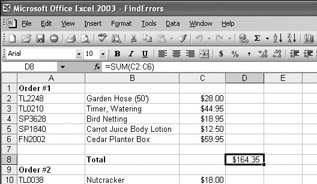Clicking cell D8, copying the cell s contents, and then pasting the result into cell D16 writes =SUM(C10:C14) into cell D16. Excel has reinterpreted the formula so that it fits the surrounding cells! Excel knows it can reinterpret the cells used in the formula because the formula uses a relative reference , or a reference that can change if the formula is copied to another cell. Relative references are written with just the cell row and column (for example, C14 ). If you want a cell reference to remain constant when the formula using it is copied to another cell, you can use an absolute reference. To write a cell reference as an absolute reference, you type \$ before the row name and the column number. If you wanted the formula in cell D16 to show the sum of values in cells C10 through C14 regardless of the cell into which it is pasted, you would write the formula as =SUM(\$C\$10:\$C\$14) .

 Tip If you copy a formula from the formula bar, use absolute references, or use only named ranges in your formula. Excel won t change the cell references when you copy your formula to another cell.

In this exercise, you create a formula to find the total cost of an order, copy that formula to another cell, and then create a formula to find the average cost of items in the order. The cells with the cost of products in this order are stored in the named range OrderItems .

USE the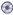Formula.xls document in the practice file folder for this topic. This practice file is located in theMy Documents\Microsoft Press\Office 2003 SBS\PerformingCalculations folder, and can also be accessed by clicking Start/All Programs/Microsoft Press/Microsoft Office System 2003 Step by Step .

OPEN theFormula.xls document.

1. Click cell D7.

D7 becomes the active cell.

2. In the formula bar, type =SUM , drag from cell D4 to D5, and press [ENTER].

The value \$63.90 appears in cell D7.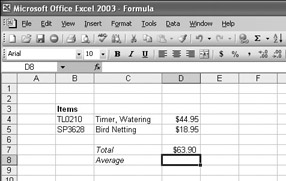3. Click cell D7, and then, on the Standard toolbar, click the Copy button.

Excel copies the formula in cell D7 to the Clipboard.

4. Click cell D8, and then, on the Standard toolbar, click the Paste button.

The value \$18.95 appears in cell D8, and =SUM(D5:D6) appears in the formula bar.

5. Press [DEL].

The formula in cell D8 disappears.

6. On the Insert menu, click Function .

The Insert Function dialog box appears.

7. Click AVERAGE , and then click OK .

The Function Arguments dialog box appears, with the contents of the Number 1 box highlighted.

8. Type OrderItems , and then click OK .

The Function Arguments dialog box disappears, and \$31.95 appears in cell D8.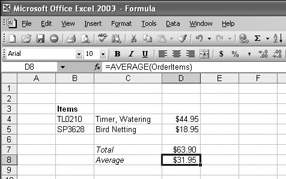9. Click cell C10.

10. On the Insert menu, click Function .

The Insert Function dialog box appears.

11. In the Select a function list, click IF and then click OK .

The Function Arguments dialog box appears.

12. In the Logical_test box, type D7 > 50 .

13. In the Value_if_true box, type "5% discount" .

14. In the Value_if_false box, type "No discount" and then click OK .

The Function Arguments dialog box disappears, and 5% discount appears in cell C10.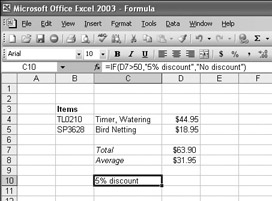15. On the Standard toolbar, click the Save button.

Excel saves your changes.

CLOSE theFormula.xls document.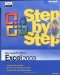MicrosoftВ® Office ExcelВ® 2003 Step by Step (Step By Step (Microsoft))
ISBN: 0735615187
EAN: 2147483647
Year: 2005
Pages: 350
Authors: Curtis Frye

Similar book on Amazon

flylib.com © 2008-2017.
If you may any questions please contact us: flylib@qtcs.net